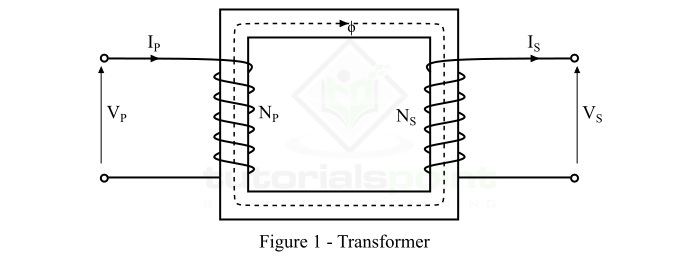# Difference between Step-Up and Step-Down Transformer

## What is a Transformer?

A transformer is an electrical device whose main function is to transmit alternating current between two circuits at a constant value of frequency, but at varying voltage levels. A transformer is constructed by combining two kinds of coil. The first coil carrying the magnetic flux is called the primary coil, while the second coil placed opposite to it, in which electromotive force (E.M.F) is induced is called as the secondary coil.

Figure-1 illustrates a standard representation of a transformer. Input voltage at the primary side of the transformer produces the varying magnetic flux on the primary coil. While the varying magnetic flux induces EMF in the secondary coil. Based on the magnitude of voltage carried by the transformer, it is divided into two categories, i.e. step-up transformer and step down transformer, which is discussed in the following sections.The variables shown in Figure-1 above is defined as follows −

• NP = Total number of turns in the primary winding,

• NS = Total number of turns in the secondary winding,

• VP = Voltage applied to the primary winding,

• VS = Voltage output from the secondary winding,

• IP = Primary winding current,

• IS = Secondary winding current,

• Φ = Magnetic flux flowing through the transformer core.

## What is a Step-Up Transformer?

A Step-Up Transformer is a kind of transformer which increases the magnitude of output voltage, while keeping a low current magnitude. The secondary winding of the step-up transformer has greater number of turns as compared to the primary winding. The main function of the step-up transformer is to transform lower voltage and high current received or induced at the primary coil of the transformer, to higher voltage and lower current at the secondary coil of the transformer.

The step-up transformer finds its application in transmitting power at longer distances wherein it transmits large amount of power at high voltages. Figure-2 illustrates the symbolic representation of the step-up transformer. The following expression shows the relation between coil turns and voltage values in the primary and secondary coils.$$\mathrm{Turns \: Ratio,K=\frac{N_{1}}{N_{2}}=\frac{V_{1}}{V_{2}}=\frac{I_{2}}{I_{1}}}$$

And,

$$\mathrm{N_{2}> N_{1};V_{2}> V_{1};I_{2}< I_{1}}$$

Where,

• N1 = Number of turn in primary winding,

• N2 = Number of turns in secondary winding,

• V1 = Primary side input voltage,

• V2 = Secondary side output voltage

The above relationship is called as the Turns Ratio, which is used to determine whether the transformer is a step-up or step-down transformer

## What is a Step-Down Transformer?

A Step-Down Transformer is a kind of transformer which reduces the magnitude of output voltage, while keeping a high current magnitude. The secondary winding of the step-down transformer has lesser number of turns as compared to the primary winding. The main function of the step-down transformer is to transform higher voltage and lower current received or induced at the primary coil of the transformer, to lower voltage and higher current at the secondary coil of the transformer.

The step-up transformer finds its application in transmitting power at shorter distances wherein the safety of the measuring instruments, home appliances or industrial equipment are considered. Figure-3 illustrates the symbolic representation of the step-down transformer.

The following expression shows the relation between coil turns and EMF values in the primary and secondary coils.For the step-down transformer, we have,

$$\mathrm{N_{2}< N_{1};V_{2}< V_{1}; I_{2}> I_{1}}$$

## Difference between Step-Up and Step-Down Transformer

The major differences between the step-up transformer and step-down transformer are described in the table given below −

Parameter

Step-Up Transformer

Step-Down Transformer

Definition

Step-up transformer converts low voltage to higher output voltage at constant frequency.

Step-down transformer converts high voltage to low output voltage at constant frequency.

Magnitude of Voltage

Voltage at primary winding is low, while the voltage at the secondary winding is high.

Voltage at primary winding is high, while the voltage at the secondary winding is low.

Magnitude of Current

Current at primary winding is high, while the current at the secondary winding is low.

Current at primary winding is low, while the current at the secondary winding is high.

Number of Turns

The primary coil is comprised of less number of turns in comparison to the secondary winding.

The secondary coil is comprised of less number of turns in comparison to the primary winding.

Standard Output Voltage Values

The output voltage generated at the output of the step-up transformer is 3.3 kV, 6.6 kV, 11 kV or greater.

The output voltage generated at the output of the step-down transformer is 10V, 20V, 110V, 220 V, 440 V, etc.

Dimension of the Transformer Windings

The primary winding is larger in size as it is made up of thick insulated copper wiring.

The secondary winding is larger in size as it is made up of thick insulated copper wiring.

Expression or Relation

The relationship between primary winding and secondary winding parameters of step-up transformer is,

$$\mathrm{N_{2}> N_{1};V_{2}> V_{1}; I_{2}< I_{1}}$$

The relationship between primary winding and secondary winding parameters of step-down transformer is,

$$\mathrm{N_{2}< N_{1};V_{2}< V_{1}; I_{2}> I_{1}}$$

Applications

The step-up transformer finds its application in extracting X-rays,microwaves, ovens, inverters, power plants, substations, etc.

The step-down transformers find their applications in main adapters, CD players, welding machines, television, doorbells, distribution substations, etc.

## Conclusion

In conclusion, the major differences between step-up and step-down transformers were listed in this article. The most significant difference between them is that, the step-up transformer is used to convert low-end voltages to high end voltage, whereas, the step-down transformer is used to transform the high-end voltage to low-end voltage. Both these transformers have several residential and industrial applications as described in this article.

Updated on: 19-Apr-2023

2K+ Views